# Use the derivative f'(x) = (x - 1)(x + 1)(x + 4) to determine the local...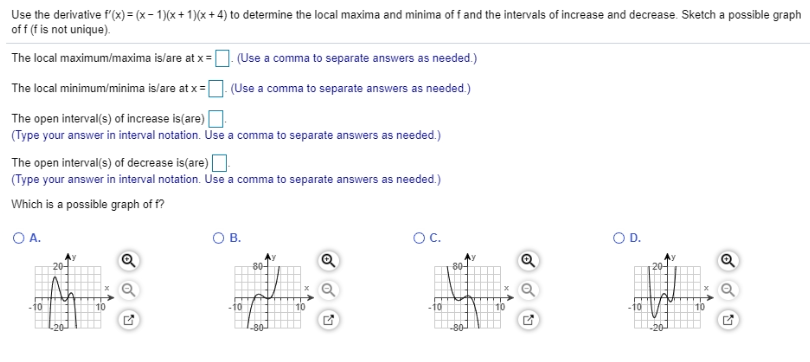Use the derivative f'(x) = (x - 1)(x + 1)(x + 4) to determine the local maxima and minima off and the intervals of increase and decrease. Sketch a possible graph off (f is not unique) The local maximum/maxima is/are at x = (Use a comma to separate answers as needed.) The local minimum/minima is/are at x = (Use a comma to separate answers as needed.) The open interval(s) of increase is(are) (Type your answer in interval notation. Use a comma to separate answers as needed.) The open interval(s) of decrease is(are) (Type your answer in interval notation. Use a comma to separate answers as needed.) Which is a possible graph of f? OA. ОВ. OC. a 20- Q 80- 80 10 - 10 - 10 10 -80 20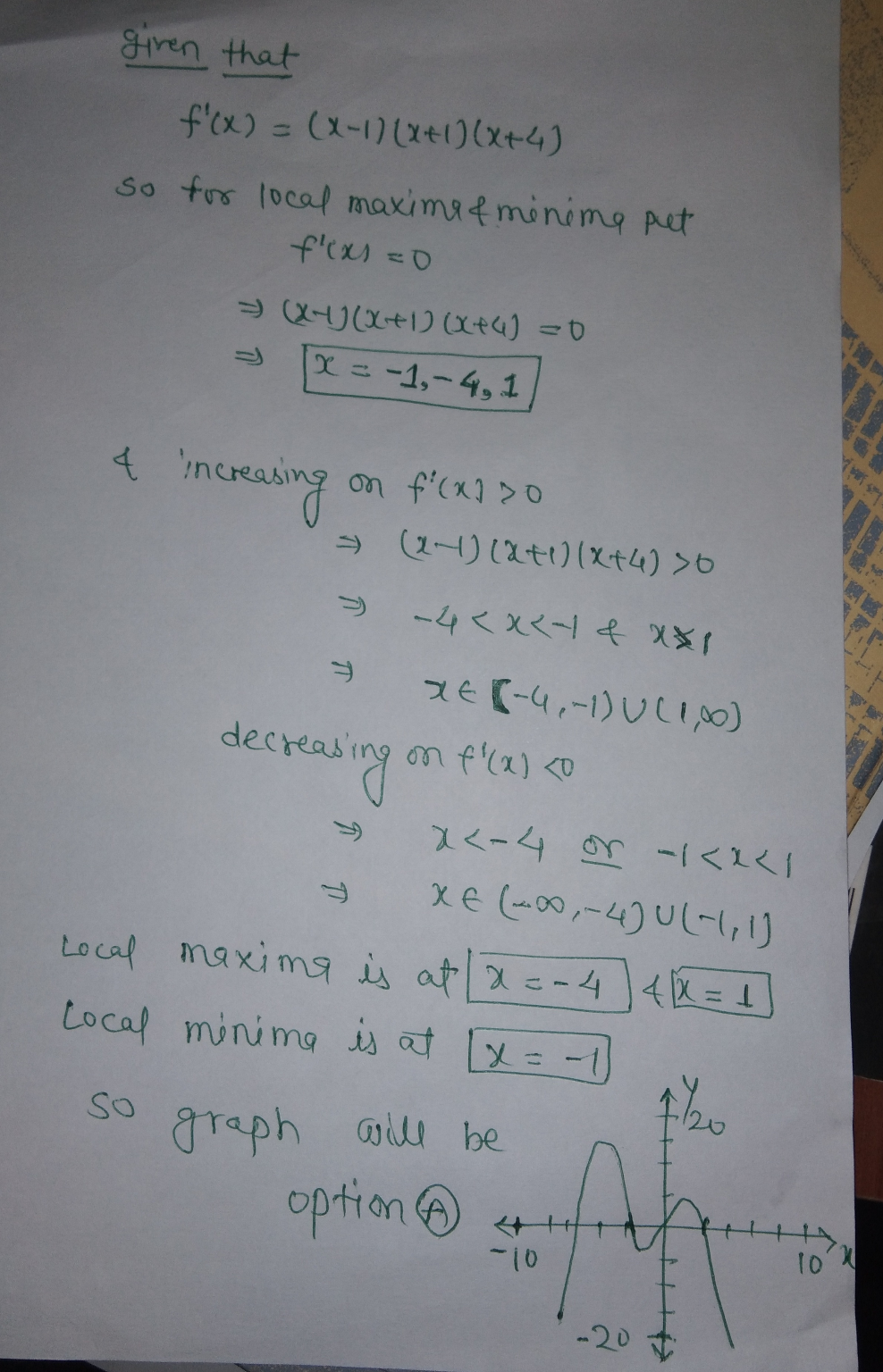#### Earn Coin

Coins can be redeemed for fabulous gifts.

Similar Homework Help Questions
• ### Use the derivative f' to determine the local minima and maxima off and the intervals of...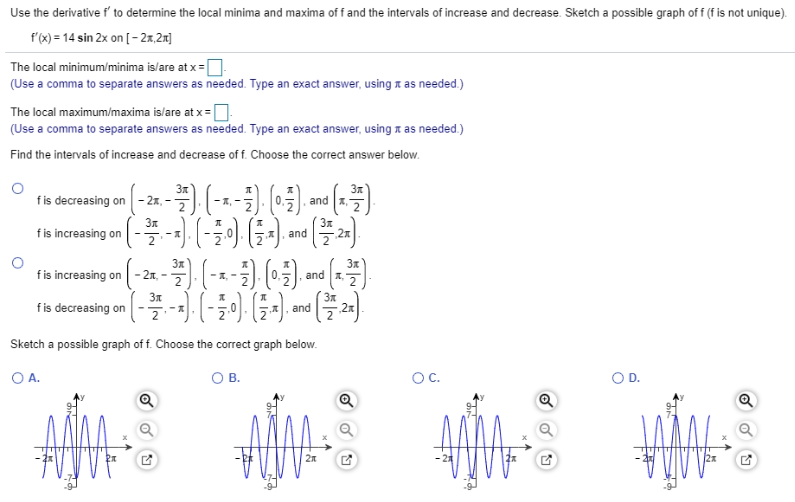Use the derivative f' to determine the local minima and maxima off and the intervals of increase and decrease. Sketch a possible graph off (f is not unique). f'(x) = 14 sin 2x on [-27,21] The local minimum/minima is/are at x = (Use a comma to separate answers as needed. Type an exact answer, using as needed.) The local maximum/maxima is/are at x = (Use a comma to separate answers as needed. Type an exact answer using t as needed.)...

• ### Locate the critical points of the following function. Then use the Second Derivative Test to determine...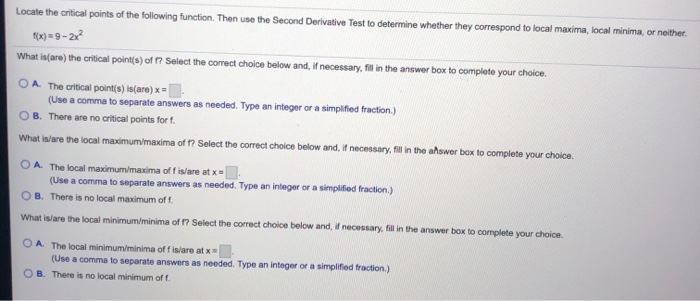Locate the critical points of the following function. Then use the Second Derivative Test to determine whether they correspond to local maxima, local minima, or neither. = 9-22 What isare) the critical point(s) of ? Select the correct choice below and, if necessary, fill in the answer box to complete your choice. O A. The critical point(s) is(are) x = (Use a comma to separate answers as needed. Type an integer or a simplified fraction) B. There are no critical...

• ### Answer the following questions about the function whose derivative is f'(x) = 2x(x - 5), a....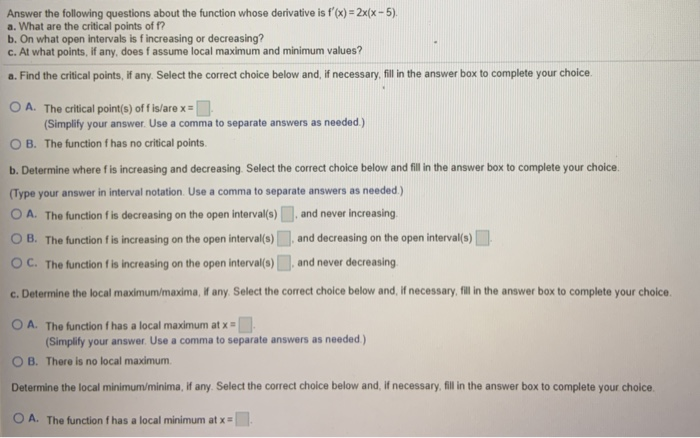Answer the following questions about the function whose derivative is f'(x) = 2x(x - 5), a. What are the critical points of f? b. On what open intervals is fincreasing or decreasing? c. At what points, if any, does fassume local maximum and minimum values? a. Find the critical points, if any. Select the correct choice below and, if necessary, fill in the answer box to complete your choice O A. The critical point(s) of fis/are x = (Simplify your...

• ### Use implicit differentiation to find y' and evaluate y'at (-1,-6). 9xy + y-48 = 0 y'...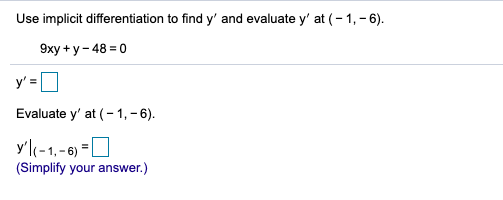Use implicit differentiation to find y' and evaluate y'at (-1,-6). 9xy + y-48 = 0 y' = Evaluate y' at (-1,-6). y'l(-1,-6)=0 (Simplify your answer.) Find the intervals on which f(x) is increasing, the intervals on which f(x) is decreasing, and the local extrema. f(x) = x + 10x + 21 Select the correct choice below and, if necessary, fill in the answer box to complete your choice. O A. The function is increasing on (Type your answer using interval...

• ### Find all critical numbers of the function f(x) = (x - 9). Then use the second-derivative...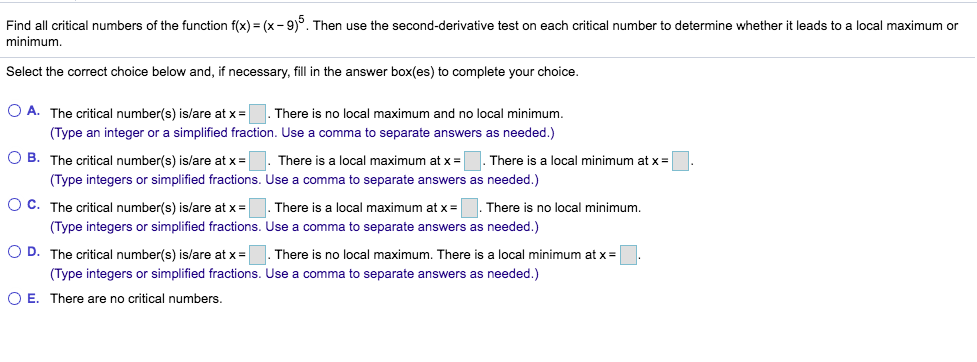Find all critical numbers of the function f(x) = (x - 9). Then use the second-derivative test on each critical number to determine whether it leads to a local maximum or minimum Select the correct choice below and, if necessary, fill in the answer box(es) to complete your choice. O A. The critical number(s) is/are at x = There is no local maximum and no local minimum. (Type an integer or a simplified fraction. Use a comma to separate answers...

• ### Use the first derivative test to determine the location of each local extremum and the value...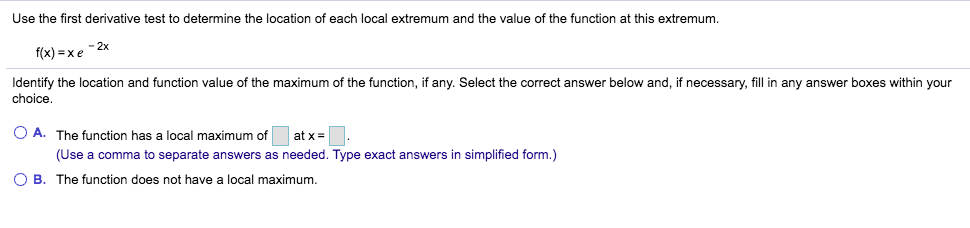Use the first derivative test to determine the location of each local extremum and the value of the function at this extremum. - 2x f(x) = x 6 Identify the location and function value of the maximum of the function, if any. Select the correct answer below and, if necessary, fill in any answer boxes within your choice. O A. The function has a local maximum of at x = (Use a comma to separate answers as needed. Type exact...

• ### U17 Find the local maxima and local minima of the function shown below. f(x,y)=x² + y2...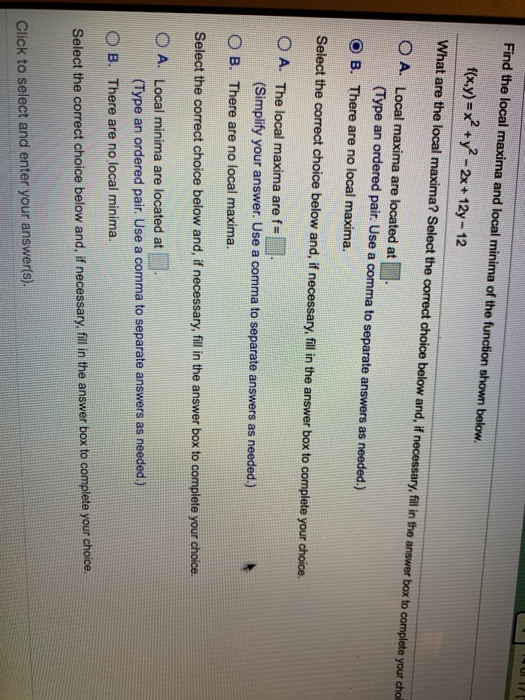U17 Find the local maxima and local minima of the function shown below. f(x,y)=x² + y2 - 2x + 12y - 12 What are the local maxima? Select the correct choice below and, if necessary, fill in the answer box to complete your choi O A. Local maxima are located at (Type an ordered pair. Use a comma to separate answers as needed.) B. There are no local maxima. Select the correct choice below and, if necessary, fill in the...

• ### Find the critical points of the following function. Use the Second Derivative Test to determine if...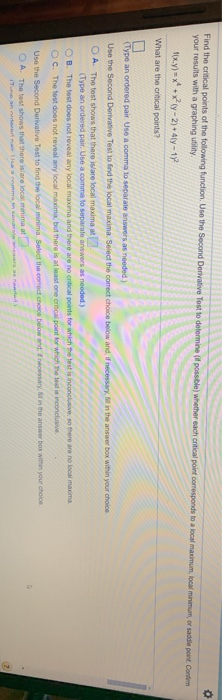Find the critical points of the following function. Use the Second Derivative Test to determine if possible) whether each critical point corresponds to a local maximum, local minimum or saddle point. Contem your results with a graphing utility f(x,y) = x + xy-2) + 4y - 12 What are the critical points? (Type an ordered pair Use a comma to separate answers as needed.) Use the Second Derivative Test to find the local maxima. Select the correct choice below and,...

• ### Find the intervals on which f(x) is increasing and the intervals on which f(x) is decreasing....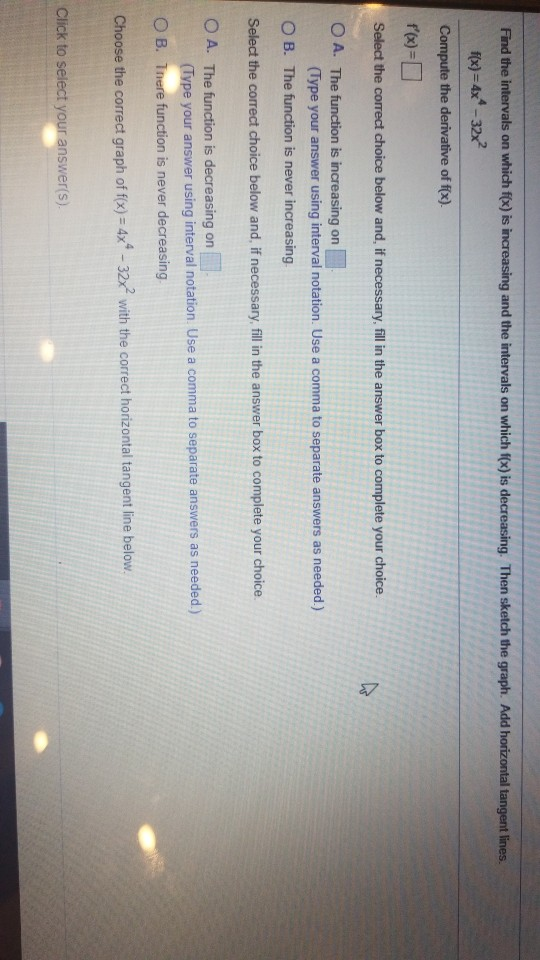Find the intervals on which f(x) is increasing and the intervals on which f(x) is decreasing. Then sketch the graph. Add horizontal tangent lines. f(x)=4x4 -32x2 Compute the derivative of f(x). f'(x)= Select the correct choice below and, if necessary, fill in the answer box to complete your choice. O A. The function is increasing on (Type your answer using interval notation. Use a comma to separate answers as needed.) OB. The function is never increasing Select the correct choice...

• ### Determine the location of each local extremum of the function. f(x) = -x2 – 3x2 -2...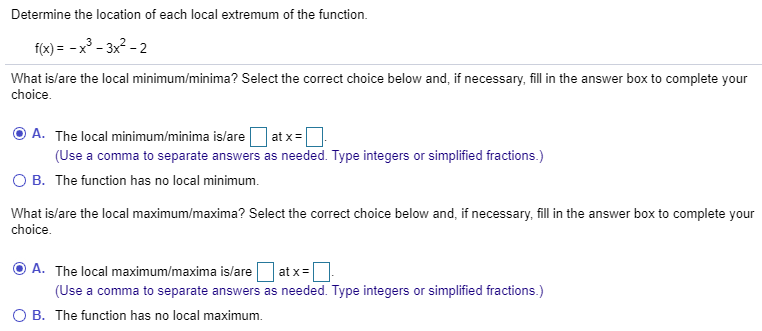Determine the location of each local extremum of the function. f(x) = -x2 – 3x2 -2 What is/are the local minimum/minima? Select the correct choice below and, if necessary, fill in the answer box to complete your choice. A. The local minimum/minima is/are atx= (Use a comma to separate answers as needed. Type integers or simplified fractions.) OB. The function has no local minimum. What is/are the local maximum/maxima? Select the correct choice below and, if necessary, fill in the...